# Area of Parallelograms, Trapezoids, and Rectangles Lesson Plan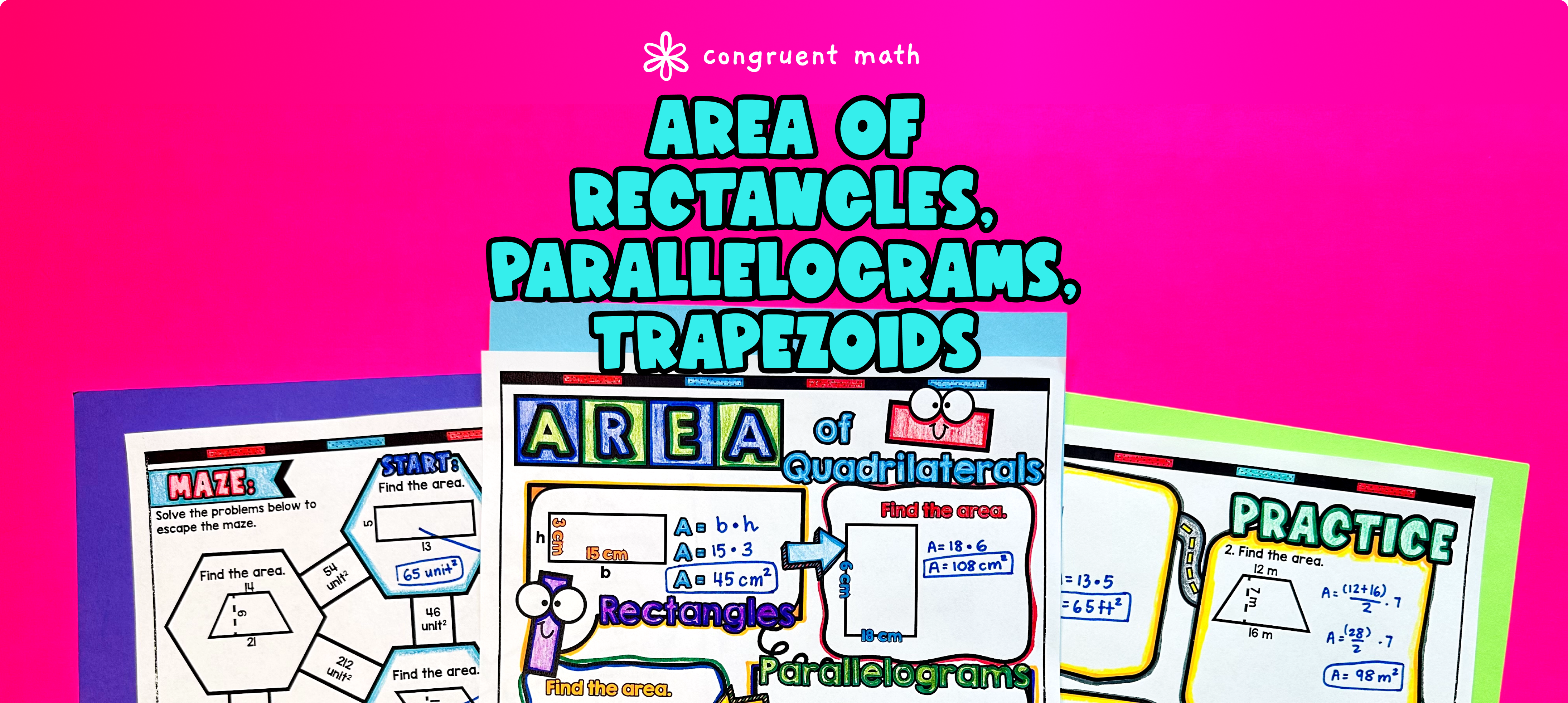Pin This

## Overview

Ever wondered how to teach the area of parallelograms, trapezoids, and rectangles in an engaging way to your middle school students?

In this lesson plan, students will learn about finding the area of these polygons and their real-life applications. Through artistic, interactive guided notes, check for understanding questions, a practice coloring worksheet, and a maze activity, students will gain a comprehensive understanding of finding the area of parallelograms, trapezoids, and rectangles.

## Get the Lesson Materials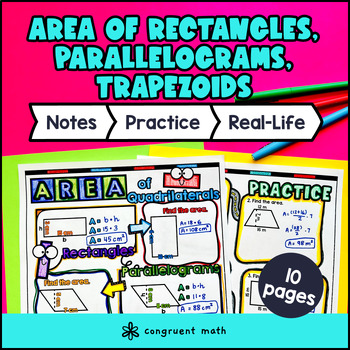\$4.25

## Learning Objectives

After this lesson, students will be able to:

• Calculate the area of parallelograms, trapezoids, and rectangles using the appropriate formulas

• Explain the real-life applications of area involving parallelograms, trapezoids, and rectangles

## Prerequisites

Before this lesson, students should be familiar with:

• How to differentiate between parallelograms, rectangles, and trapezoids

• Knowledge of basic multiplication and addition skills for whole numbers

• Understanding of basic algebraic concepts, such as substituting values into formulas

## Key Vocabulary

• Parallelogram

• Trapezoid

• Rectangles

## Procedure

### Introduction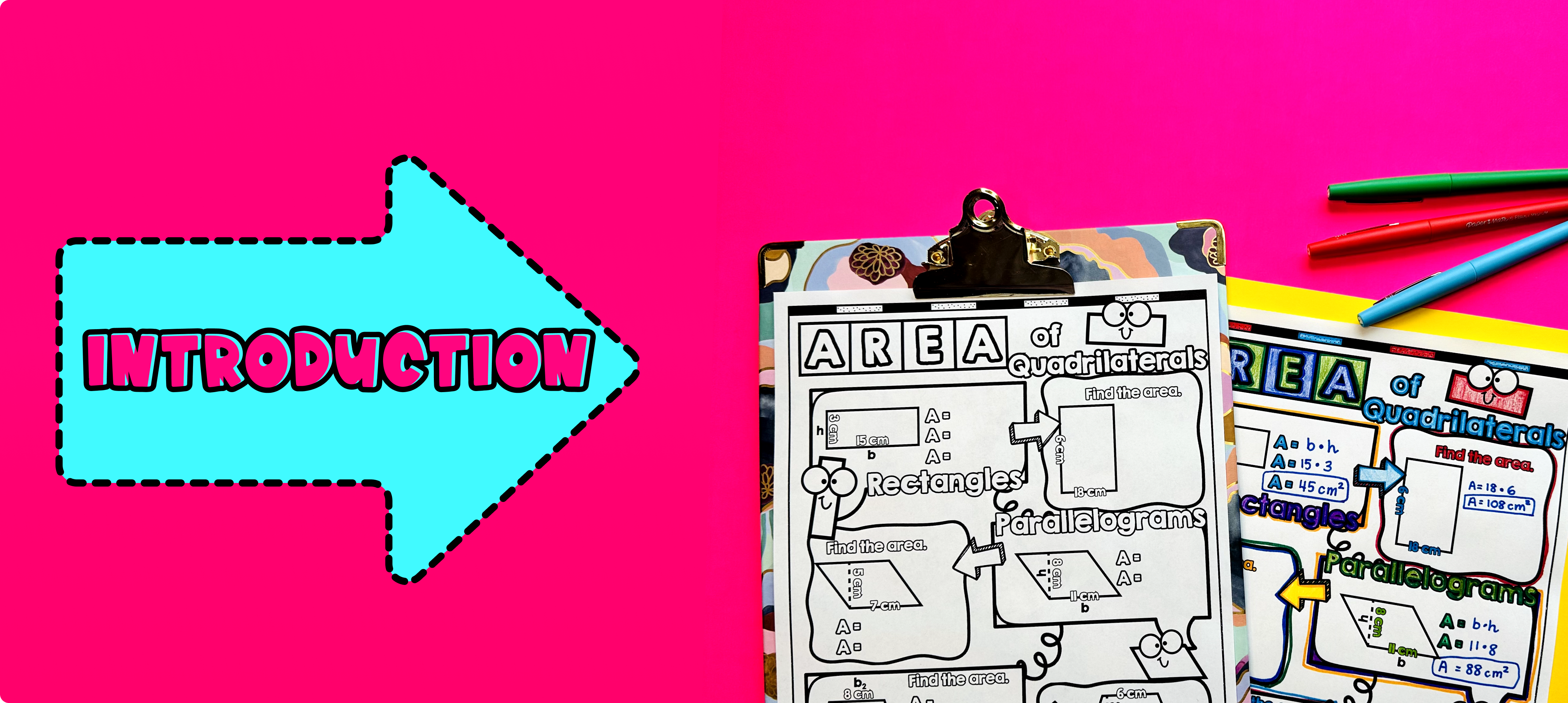Pin This

As a hook, ask students why it is important to calculate the area of different polygons, specifically quadrilaterals, in real-life situations. Refer to the last page of the guided notes as well as the FAQs below for ideas.

Use the first page of the guided notes to introduce the formulas for finding the area of rectangles, parallelograms, and trapezoids. Walk through the key points of finding the length and width of a rectangle and using the formula A = l * w to calculate its area. Then, walk through the key points of identifying the base and height of a parallelogram and using the formula A = b * h to find its area. Then, walk through the key points of identifying the bases, height, and average of the bases of a trapezoid, and using the formula A = (b1 + b2) * h / 2 to calculate its area. For each polygon, students will take notes on the formula and also practice an example for a rectangle, parallelogram, and trapezoid.

Based on student responses, reteach concepts that students need extra help with. If your class has a wide range of proficiency levels, you can pull out students for reteaching, and have more advanced students begin work on the practice exercises.

### Practice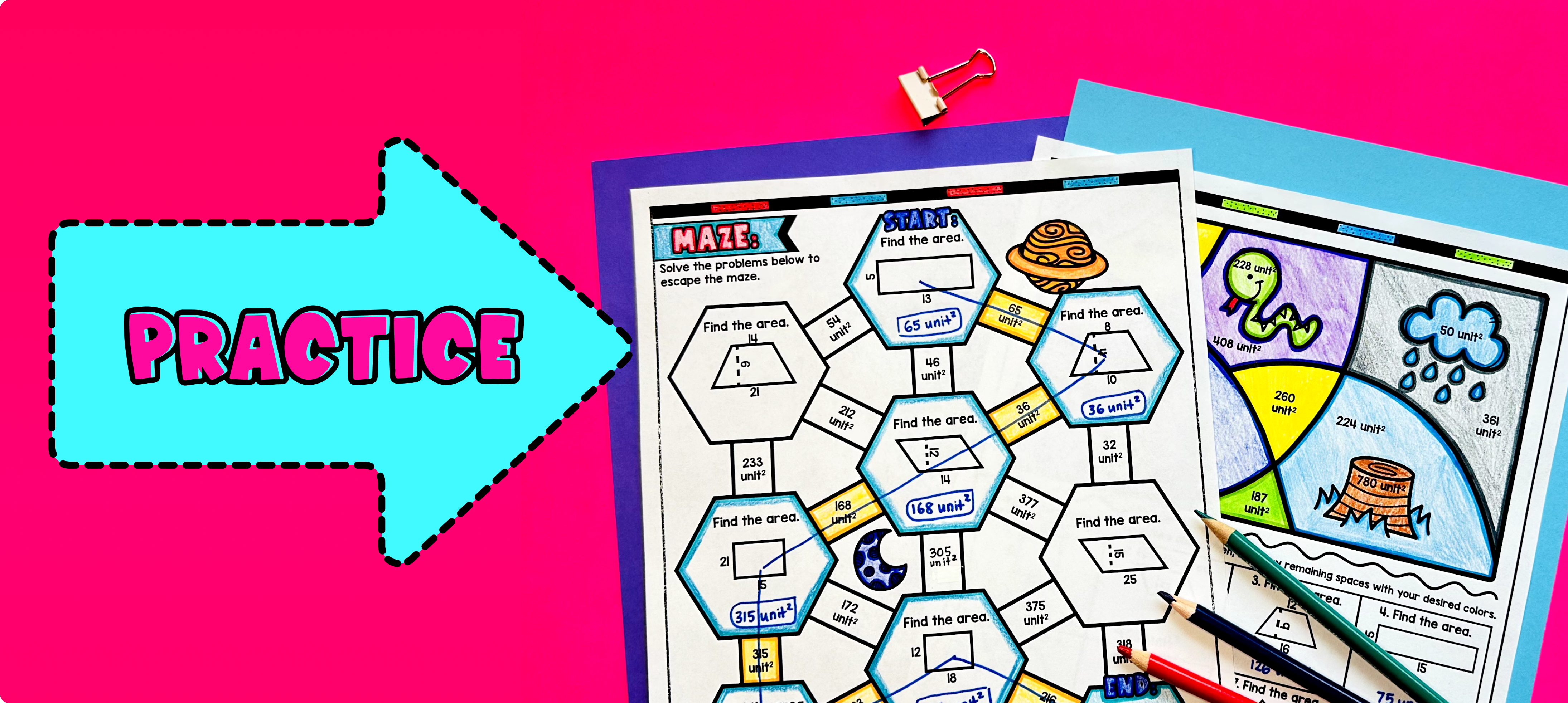Pin This

Have students practice finding the area of parallelograms, trapezoids, and rectangles using the practice sheet on page 2. This activity allows students to apply the concepts they learned in the guided notes and practice calculating the area of different polygons.

Walk around the classroom to answer any student questions and provide individual support as needed. Fast finishers can dive into the color by number and maze activity for extra practice. This maze requires students to calculate the area of different shapes and find the correct path to reach the end. You can assign it as homework for the remainder of the class to reinforce their understanding of finding the area of parallelograms, trapezoids, and rectangles.

### Real-Life Application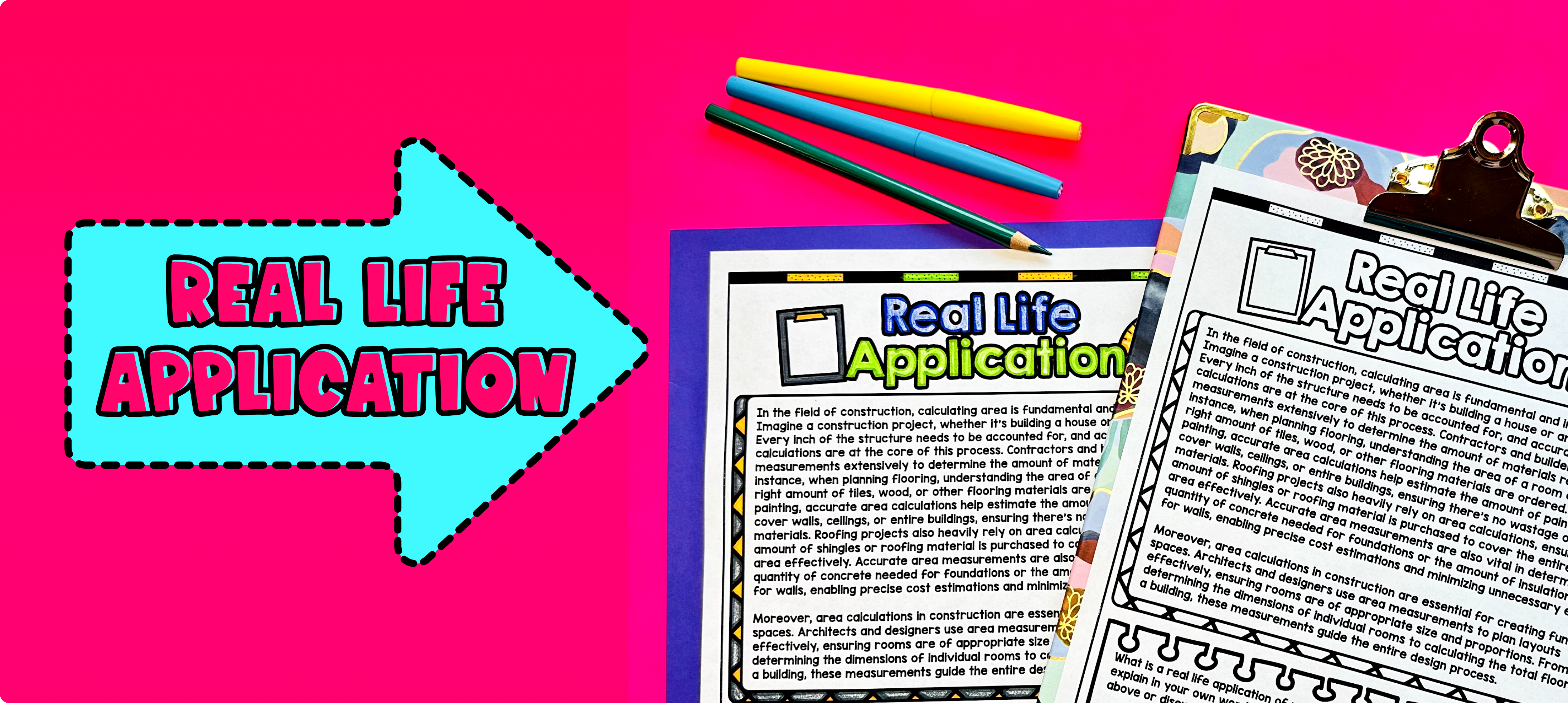Pin This

Use the last page of the guided notes "real life applications" to bring the class back together, and introduce the concept of real-life applications of calculating the area of different polygons, specifically quadrilaterals. Explain to students that understanding how to find the area of shapes can be useful in many real-life situations.

Ask students if they can think of any real-life situations where knowing how to calculate area would be important. Encourage students to share their ideas and write them on the board. Some examples may include:

• Carpeting a room: Knowing the area of a room can help determine how much carpet is needed.

• Painting a wall: Calculating the area of a wall can help determine how much paint is needed.

• Gardening: Finding the area of a garden bed can help determine how much soil or mulch is needed.

• Building a fence: Understanding the area can help determine how much material is needed to build a fence.

Refer to the Frequently Asked Questions (FAQ) section for additional ideas on how to teach real-life applications of finding the area of polygons.

## Extensions

If you’re looking for digital practice for area of parallelograms, trapezoids, and rectangles, try my Pixel Art activities in Google Sheets. Every answer is automatically checked, and correct answers unlock parts of a mystery picture. It’s incredibly fun, and a powerful tool for differentiation.

Here is an activity to explore: